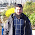# Excel : Intersection between curve and straight line

To find intersection of curve and a straight line we first need to know the mathematical condition behind it.

When two lines cross:
1. Before intersection, value of y1 is less than y2 at given value of x
2. After intersection, value of y1 is less than y2 at next level of x value (xi+1)

I consider two equations : y1=2x+5  and y2=x^2+1 . On the basis of these equations i created data for three variables that are x, y1 and y2.

To find the intersection point :

STEP I

Calculate the difference between y1 and y2 at every level of x

STEP II

Compare the values of Y for the two lines in every successive row.

=IF(SIGN(F6*F7)=1,0,1)

STEP III:

Identify the x values between which the lines have crossed

=MATCH(1,G6:G15,0)

STEP IV:

Calculate X's and Y's data points

=INDEX(C\$6:C\$15,H6)

STEP V:

Find slope and intercept using the above data points

=SLOPE(J\$6:J\$7,\$I\$6:\$I\$7)

=INTERCEPT(J\$6:J\$7,\$I\$6:\$I\$7)

STEP VI:

To find the X-point at which  Y1<Y2 beomes Y2<Y1 :
y1=m1x1+c1 and y2=m2x2+c2
Where m1: Gradient or Slope of line1, c1: Intercept of line1, m2: Gradient or Slope of line2, c2: Intercept of line2.

Since at the point of intersection, the two equations (form y=mx+c) will have the same values of x and y, we set the two equations equal to each other. This gives an equation that we can solve for x .We substitute that x value in one of the line equations and solve it for y

Y= (c2*m1-c1*m2)/(m1-m2) ,

X=(Y-m2)/c2

STEP VII:

Insert Scatter with Straight lines chart and mark intersection point
( Right click on lines >> Format Data series >> Marker Options / Fill )
Click on the link below to download the excel file
Intersection between lines

Related link : Intersection of two linear straight linesRelated PostsDeepanshu founded ListenData with a simple objective - Make analytics easy to understand and follow. He has over 8 years of experience in data science. During his tenure, he has worked with global clients in various domains like Banking, Insurance, Telecom and Human Resource.

5 Responses to "Excel : Intersection between curve and straight line"
1.This only works if the intersection points are exactly alike and not if a line is interpolated. Just my 2 cents

2.What if I don't know the equation of the curve and the graph is generated through given data points?

3.Simply Marvelous! Excellent Job

4.Thank a lot!

5.Thanks Mate. Helped!

Next → ← Prev
Love this Post? Spread the Word!
Share